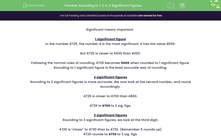# Practise Rounding to 1, 2 or 3 Significant Figures

In this worksheet, students will practise rounding to 1, 2 or 3 significant figures.Key stage:  KS 3

Curriculum topic:   Number

Curriculum subtopic:   Round/Approximate Numbers Appropriately

Popular topics:   Rounding worksheets, Place Value worksheets

Difficulty level:#### Worksheet Overview

This activity is all about significant figures.

Significant means important.

1 significant figure

In the number 4,725, the number 4 is the most significant. It has the value 4,000.

But 4,725 is closer to 5,000 than 4,000.

Following the normal rules of rounding, 4,725 becomes 5,000 when rounded to 1 significant figure. Rounding to 1 significant figure is the least accurate way of rounding.

2 significant figures

Rounding to 2 significant figures is more accurate. We now look at the second number, and round accordingly.

4,725 is closer to 4,700 than 4,800.

4,725 is 4,700 to 2 sig. figs.

3 significant figures

Rounding to 3 significant figures, we look at the third digit.

4,725 is closer to 4,730 than to 4,720. (Remember 5 rounds up)

4,725 rounds to 4,730 to 3 sig. figs.

Common mistake

It is a common mistake to say that 4,725 rounds to 5 to 1 sig. fig., instead of 5,000.

The zeros here are important to get the place values correct. They are important but not significant.

Let's try some questions now.

### What is EdPlace?

We're your National Curriculum aligned online education content provider helping each child succeed in English, maths and science from year 1 to GCSE. With an EdPlace account you’ll be able to track and measure progress, helping each child achieve their best. We build confidence and attainment by personalising each child’s learning at a level that suits them.

Get started